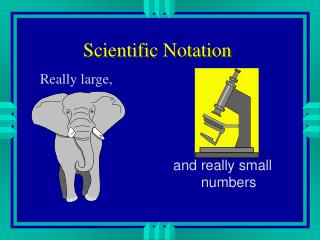DownloadDownload PresentationScientific Notation

# Scientific Notation

Télécharger la présentation## Scientific Notation

- - - - - - - - - - - - - - - - - - - - - - - - - - - E N D - - - - - - - - - - - - - - - - - - - - - - - - - - -
##### Presentation Transcript

1. Scientific Notation Really large, and really small numbers

2. Why? • Chemistry often deals with very large and very small numbers. • There are 602,000,000,000,000,000,000,000 molecules of water in 18 mL • one electron has a mass of 0.000000000000000000000000000911 g • We need a shorter way of writing these numbers

3. Standard Exponential Form • another name for scientific notation. • consists of two parts • a number between 1 and 10 • multiplied by 10, raised to some power • 602,000,000,000,000,000,000,000 = 6.02 x 1023 • 0.000000000000000000000000000911 g = 9.11 x 10-28

4. Putting a number into scientific notation • determine how many times you have to move the decimal place to make it into a number between 1 and 10 • 3240000 • use that as the power of 10 • 3.24 x 106

5. What is the number is smaller? • if you make the number bigger by moving the decimal point, make the exponent smaller and visa-versa • 0.00045 • 4.5 x 10-4

6. Getting back to original number • Move the decimal point the same number of times as the exponent • If the exponent gets bigger the number gets smaller • Change 2.99 x 108 • Change 4.87 x 10-3

7. Using your calculator • EE and EXP button stand for x 10 to the • 4.5 x 10-4 • push 4.5 • push either EXP or EE • push 4 +/- or -4 • see what your display says.

8. Practice these problems • (4.8 x 10 5 ) x (6.7 x 10-6) • Remember when you multiply you add exponents • 106x 10-4 • When you divide you subtract exponents. • (6.8 x 10 -6) • (3.2 x 10 4)

9. Adding and Subtracting • You can’t add or subtract numbers until they are to the same power of ten. • Your calculator does this automatically. • (4.8 x 10 5 ) + (6.7 x 106) • (6.8 x 10 -6) - (3.2 x 10-5) • Remember- standard form starts with a number between 1 and 10 to start.

10. PEMDAS

11. PEMDAS